Next: ROBINSON'S ENERGY DELAY THEOREM Up: Spectral factorization Previous: Spectral factorization

# ROOT METHOD

The time function (2, 1) has the same spectrum as the time function (1, 2). The autocorrelation is (2, 5, 2). We may utilize this observation to explore the multiplicity of all time functions with the same autocorrelation and spectrum. It would seem that the time reverse of any function would have the same autocorrelation as the function. Actually, certain applications will involve complex time series; therefore we should make the more precise statement that any wavelet and its complex-conjugate time-reverse share the same autocorrelation and spectrum. Let us verify this for simple two-point time functions. The spectrum of (b0, b1) is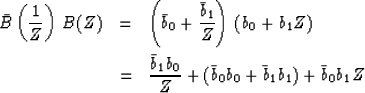(1)
The conjugate-reversed time function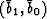with Z transform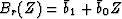has a spectrum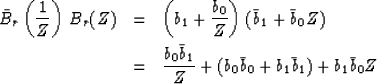(2)
We see that the spectrum (1) is indeed identical to (2). Now we wish to extend the idea to time functions with three and more points. Full generality may be observed for three-point time functions, say B(Z) = b0 + b1 Z + b2 Z2. First, we call upon the fundamental theorem of algebra (which states that a polynomial of degree n has exactly n roots) to write B(Z) in factored form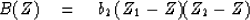(3)
Its spectrum is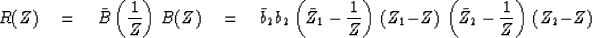(4)

Now, what can we do to change the wavelet (3) which will leave its spectrum (4) unchanged? Clearly, b2 may be multiplied by any complex number of unit magnitude. What is left of (4) can be broken up into a product of factors of form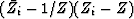.But such a factor is just like (3). The time function of (Zi - Z) is (Zi, -1), and its complex-conjugate time-reverse is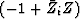.In a generalization of (3) there could be N factors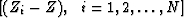.Any combination of them could be reversed. Hence there are 2N different wavelets which may be formed by reversals, and all of the wavelets have the same spectrum. Let us look off the unit circle in the complex plane. The factor (Zi - Z) means that Zi is a root of both B(Z) and R(Z). If we replace (Zi - Z) byin B(Z), we have removed a root at Zi from B(Z) and replaced it by another at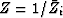.The roots of R(Z) have not changed a bit because there were originally roots at both Zi and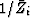and the reversal has merely switched them around. Summarizing the situation in the complex plane, B(Z) has roots Zi which occur anywhere, R(Z) must have all the roots Zi and, in addition, the roots.Replacing some particular root Zi bychanges B(Z) but not R(Z). The operation of replacing a root at Zi by one atmay be written as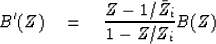(5)
The multiplying factor is none other than the all-pass filter considered in an earlier chapter. With that in mind, it is obvious that B'(Z) has the same spectrum as B(Z). In fact, there is really no reason for Zi to be a root of B(Z). If Zi is a root of B(Z), then B'(Z) will be a polynomial; otherwise it will be an infinite series.

Now let us discuss the calculation of B(Z) from a given R(Z). First, the roots of R(Z) are by definition the solutions to R(Z) = 0. If we multiply R(Z) by ZN (where R(Z) has been given up to degree N), then ZN R(Z) is a polynomial and the solutions Zi to ZN R(Z) = 0 will be the same as the solutions of R(Z) = 0. Finding all roots of a polynomial is a standard though difficult task. Assuming this to have been done we may then check to see if the roots come in the pairs Zi and.If they do not, the R(Z) was not really a spectrum. If they do, then for every zero inside the unit circle, we must have one outside. Refer to Figure 1.

 3-1 Figure 1 Roots of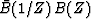.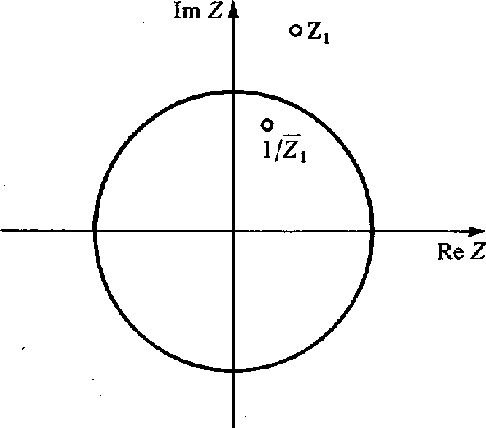Thus, if we decide to make B(Z) be a minimum-phase wavelet with the spectrum R(Z), we collect all of the roots outside the unit circle. Then we create B(Z) with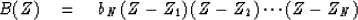(6)

This then summarizes the calculation of a minimum-phase wavelet from a given spectrum. When N is large, it is computationally very awkward compared to methods yet to be discussed. The value of the root method is that it shows certain basic principles.

1.
Every spectrum has a minimum-phase wavelet which is unique within a complex scale factor of unit magnitude.
2.
There are infinitely many time functions with any given spectrum.

3.
Not all functions are possible autocorrelation functions.

The root method of spectral factorization was apparently developed by economists in the 1920s and 1930s. A number of early references may be found in Wold's book, Stationary Time Series.

## EXERCISES:

1. How can you find the scale factor bN in (6)?
2. Compute the autocorrelation of each of the four wavelets (4, 0, -1), (2, 3, -2), (-2, 3, 2), (1, 0, -4).
3. A power spectrum is observed to fit the form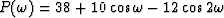.What are some wavelets with this spectrum? Which is minimum phase? (HINT: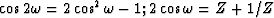;use quadratic formula.)
4. Show that if a wavelet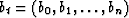is real, the roots of the spectrum R come in the quadruplets Z0, 1/Z0,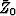, and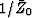.Look into the case of roots exactly on the unit circle and on the real axis. What is the minimum multiplicity of such roots?Next: ROBINSON'S ENERGY DELAY THEOREM Up: Spectral factorization Previous: Spectral factorization
Stanford Exploration Project
10/30/1997